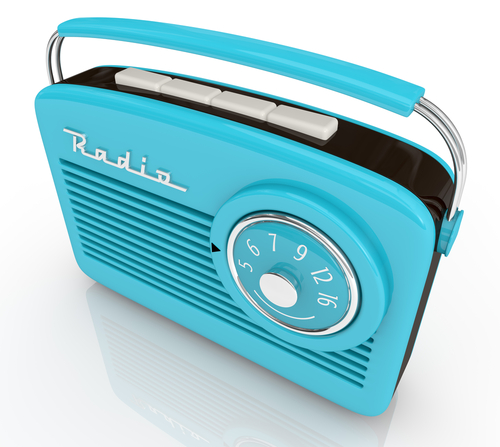# Find the Original Amount Before a Percentage Change

In this worksheet, students will apply reverse percentages to find starting amounts before a percentage increase or decrease has occurred.Key stage:  KS 4

Year:  GCSE

GCSE Subjects:   Maths

GCSE Boards:   AQA, Eduqas, Pearson Edexcel, OCR,

Difficulty level:#### Worksheet OverviewImagine that our friend bought us a birthday present in the sale, and we want to know how much the item was worth before the sale.

Or we may have missed out on a promotional offer on Black Friday, and want to know how much the price of an item has increased by.

Finding the original amount, before a percentage change took place, is also known as reverse percentages.

The key word here is reverse - remembering this is going to help us throughout this activity.

Let's look at reverse percentages in action now in some examples.e.g. A radio sells for £63, after a 40% increase in the cost price.

What was the original cost price?

We know that the radio sells for £63 after a 40% increase, so we can write this as:

c × 1.4 = 63 (where 'c' represents the original cost)

If we solve this using normal algebraic rules, we find that:

c = 63 ÷ 1.40 = £45

e.g. A packet of washing powder is advertised as having an extra 20% powder.

If it contains 1.32 kg, what amount would be in the original pack?

p × 1.20 = 1.32 kg

p = 1.32 ÷ 1.20 = 1.1 kg

e.g. A television has been reduced by 8% in a sale.

It now costs £586.

What was the cost of the television before the reduction in price?

This time, as we need to find a reduction rather than an increase, we need to take 8% away from 100% to find our multiplier:

100 - 8 = 92% or × 0.92

Now we need to apply the inverse (opposite) operation to an increase, like this:

£586 ÷ 0.92 = £636.96

Let us extend this a little more now...

You could see questions that don't appear as straightforward as these examples, and may require a different approach.

Here's an example to illustrate what we mean.e.g. At a hockey game, there are 60 children present.

This is 20% of the total amount of people at the game.

How many people are present at the game in total?

Think about what we know here:

20% = 60 children and 20% × 5 = 100%

So if we multiply 20% by 5, we reach the total amount in this case:

60 × 5 = 300 peoplee.g. In a shoe factory, 33% of the shoes are leather and the remaining 4355 are suede.

How many shoes are leather?

As we cannot easily turn 33% into 100% (as we did in the previous example), we have to adopt a different approach here.

Think about what we know again.

If 33% of the shoes are leather, the suede shoes must make up the remaining 67%, so:

4355 = 67%

To make life easier, we want to find 1%:

4355 ÷ 67 = 65

If 1% = 65, then...

33% = 65 × 33 = 2145 shoes

In this activity, we will apply reverse percentages to find starting amounts before a percentage increase or decrease has occurred, using the methods shown above.

### What is EdPlace?

We're your National Curriculum aligned online education content provider helping each child succeed in English, maths and science from year 1 to GCSE. With an EdPlace account you’ll be able to track and measure progress, helping each child achieve their best. We build confidence and attainment by personalising each child’s learning at a level that suits them.

Get started